# Swift Algorithm Club: Minimum Spanning Tree with Prim’s Algorithm

Learn how to implement a Swift minimum spanning tree using Prim’s algorithm, in this step by step tutorial.

The Swift Algorithm Club is an open source project on implementing data structures and algorithms in Swift.

Every month, Kelvin Lau, Ross O’Brien and I feature a cool data structure or algorithm from the club in a tutorial on this site. If you want to learn more about algorithms and data structures, follow along with us!

In this tutorial you will learn to implement a Swift minimum spanning tree, specifically using prim’s algorithm.

Prim’s algorithm was first implemented for the Swift Algorithm Club by Xiang Xin (thank you!), and has been presented here for tutorial format.

Note: If you have been follow along our SAC article series, last time you saw how to create a heap and priority queue. You will now use a priority queue to implement Prim’s algorithm.

## Introduction

Prim’s algorithm was first discovered by a mathematician named Vojtěch Jarník, and later again by Robert Prim. This algorithm is also known as a greedy algorithm.

A greedy algorithm is used to find optimal solutions. It constructs a solution step by step, where at every stage it picks the most optimal path.

Prim’s algorithm constructs a minimum spanning tree. A minimum spanning tree is formed by a subset of connected undirected weighted edges, that connect all vertices together without forming a cycle. Also it has the minimum possible total edge weight. For example you might want to find the cheapest way to layout your water pipes effectively, to cut cost.

In Prim’s algorithm you create a minimum spanning tree by picking edges one at a time. It’s a greedy algorithm because every time you pick an edge, you always pick the smallest weighted edge that connects a pair of vertices.

For other applications of greedy algorithm check out this link by UT Dallas.

## Getting Started

Before you start implementing prim’s algorithm, there are 6 steps to consider when performing it: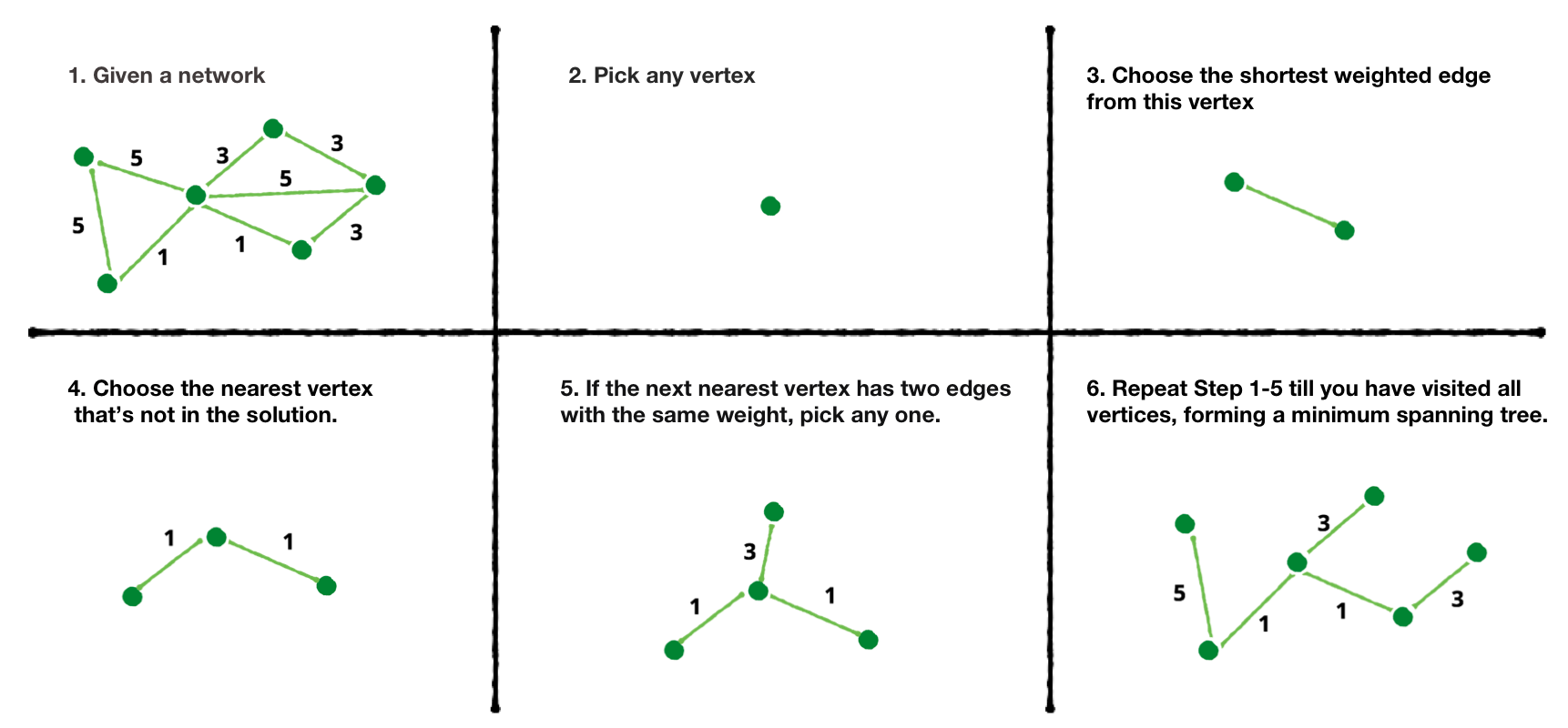Let’s go through a simple example. Imagine the network graph below. It could represent any type of network! Let’s open up our imagination here!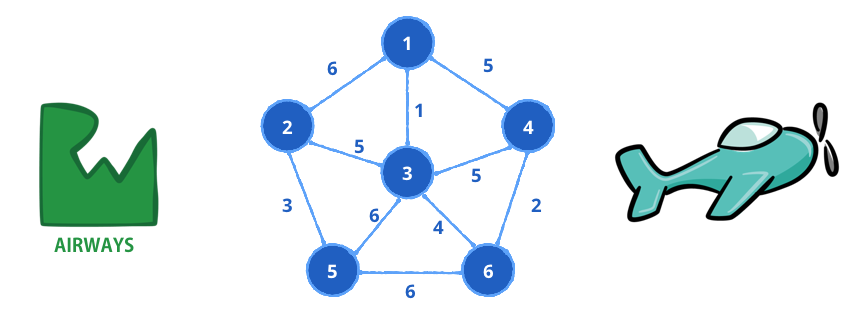Imagine a network of airports represented by the vertices. The weighted edges represents the cost and route for an airplane to fly from one airport to the next. RW Airways may want to adopt prim’s algorithm to get a minimum spanning tree giving them the most cost effective routes to fly between airports!

By the end of this tutorial you will save lots of dollar bills!## Working through an example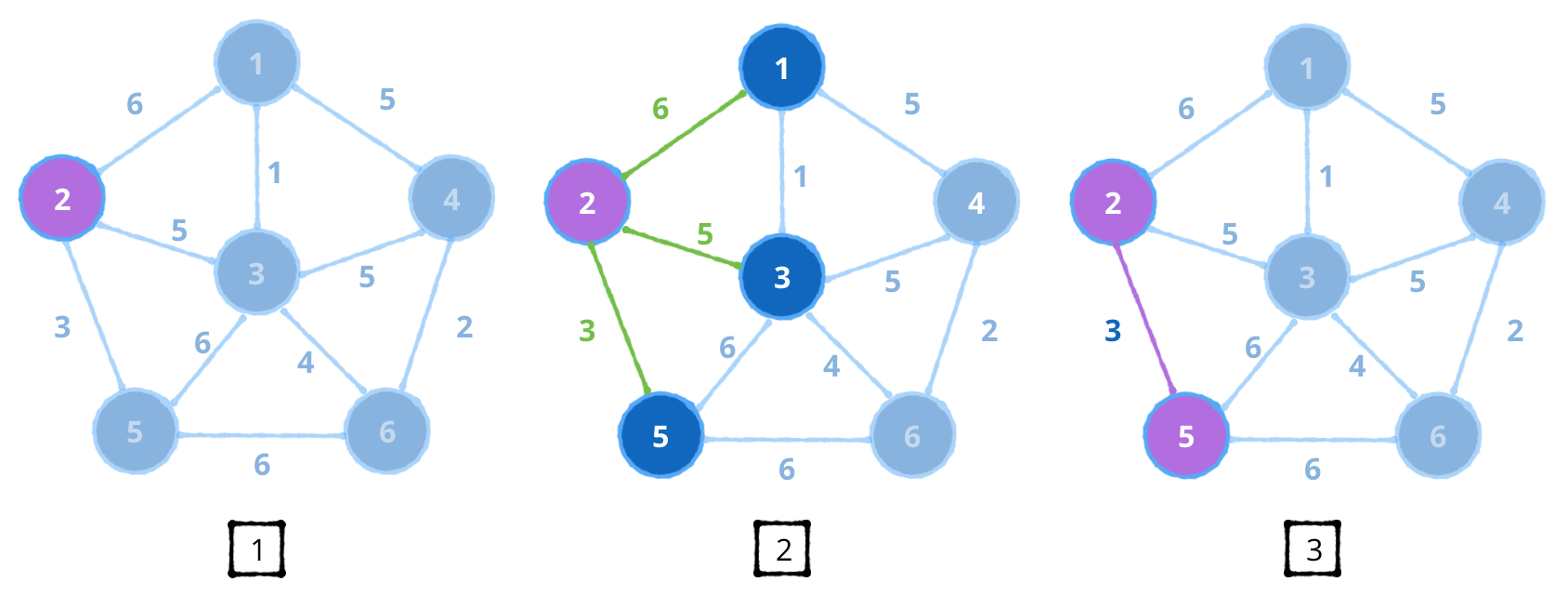1. First, start by picking any vertex. Let’s say vertex 2.
2. Choose the shortest edge from this vertex. This vertex has edges with weights { 6, 5, 3 }.
3. You pick edge 3 since it’s the smallest, which routes to vertex 5.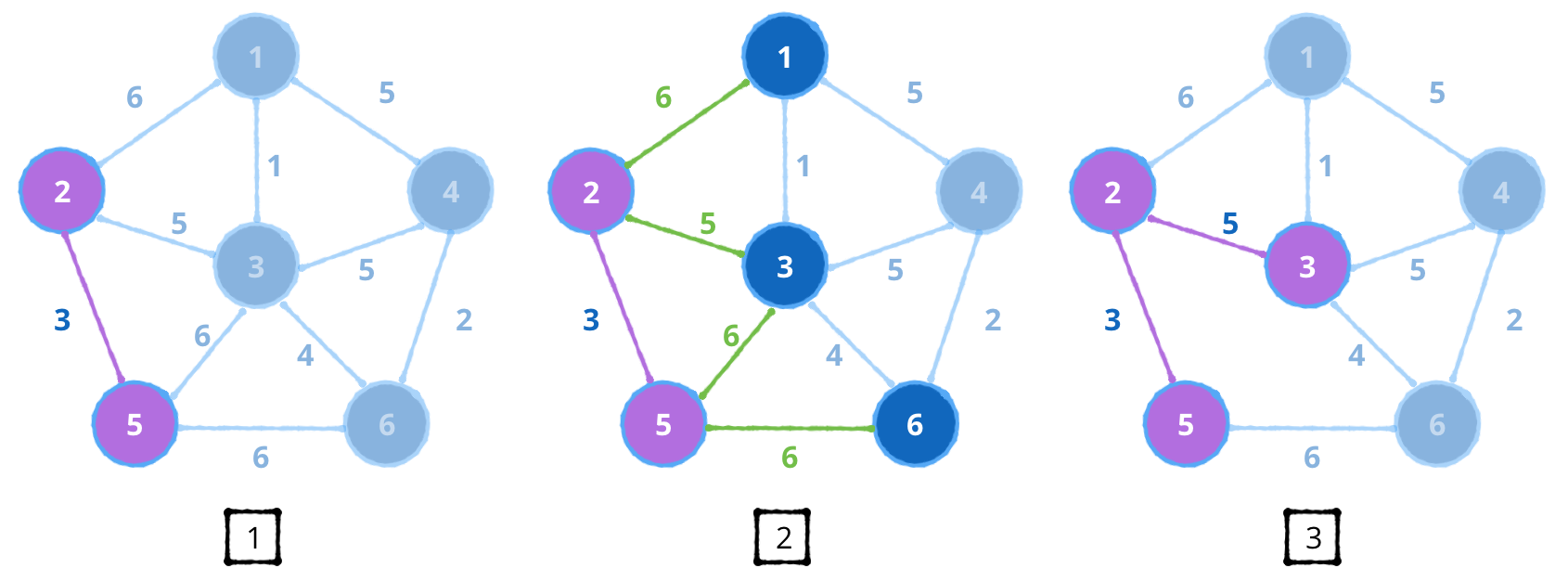1. You have now visited vertices { 2, 5 }.
2. Choose the shortest edge from these vertices. The next nearest edges are { 6, 5, 6, 6 }. You pick edge 5 since it’s the smallest, which routes to vertex 3.
3. Notice that edge 6 between vertex 5 and vertex 3 can be removed since these vertices have already been visited.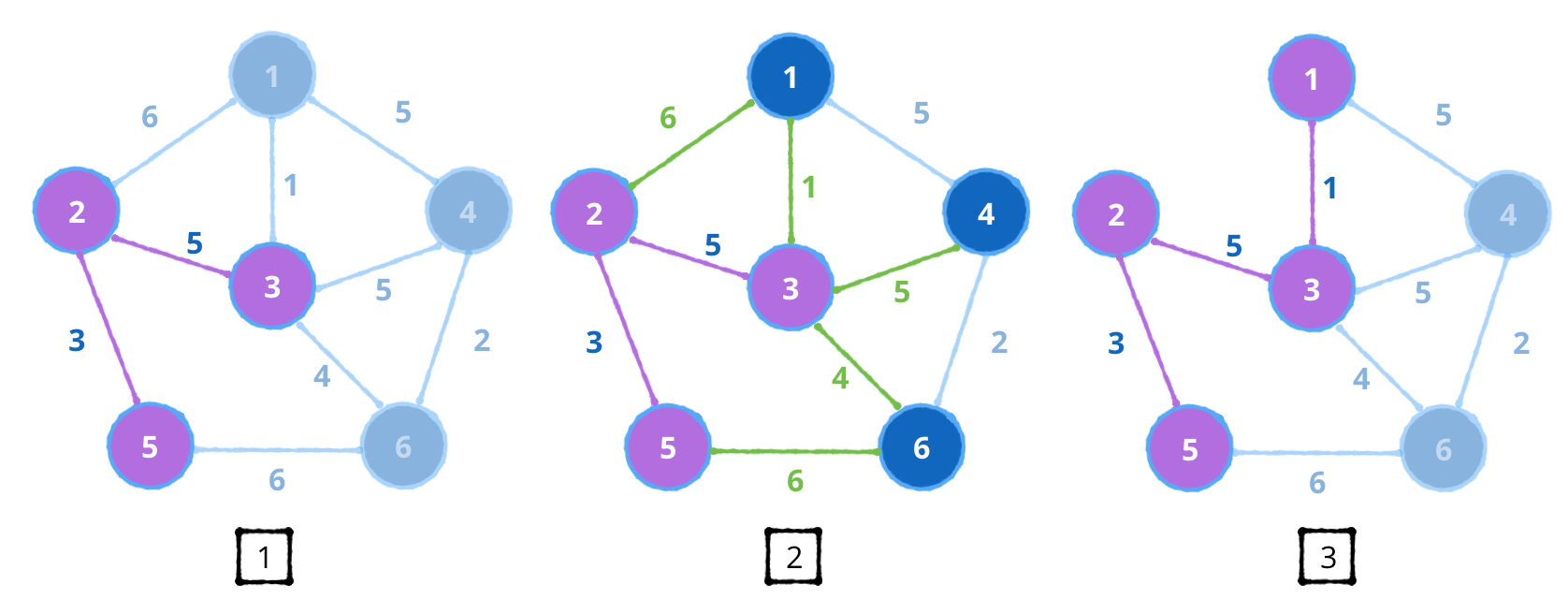1. You now have visited vertices { 2, 3, 5 } .
2. Choose the shortest edge from these vertices. The next nearest edges are { 6, 1, 5, 4, 6 } . You pick edge 1 since it’s the smallest, which routes to vertex 1.
3. Notice that edge 6 between vertex 2 and vertex 1 can be removed since these vertices have already been visited.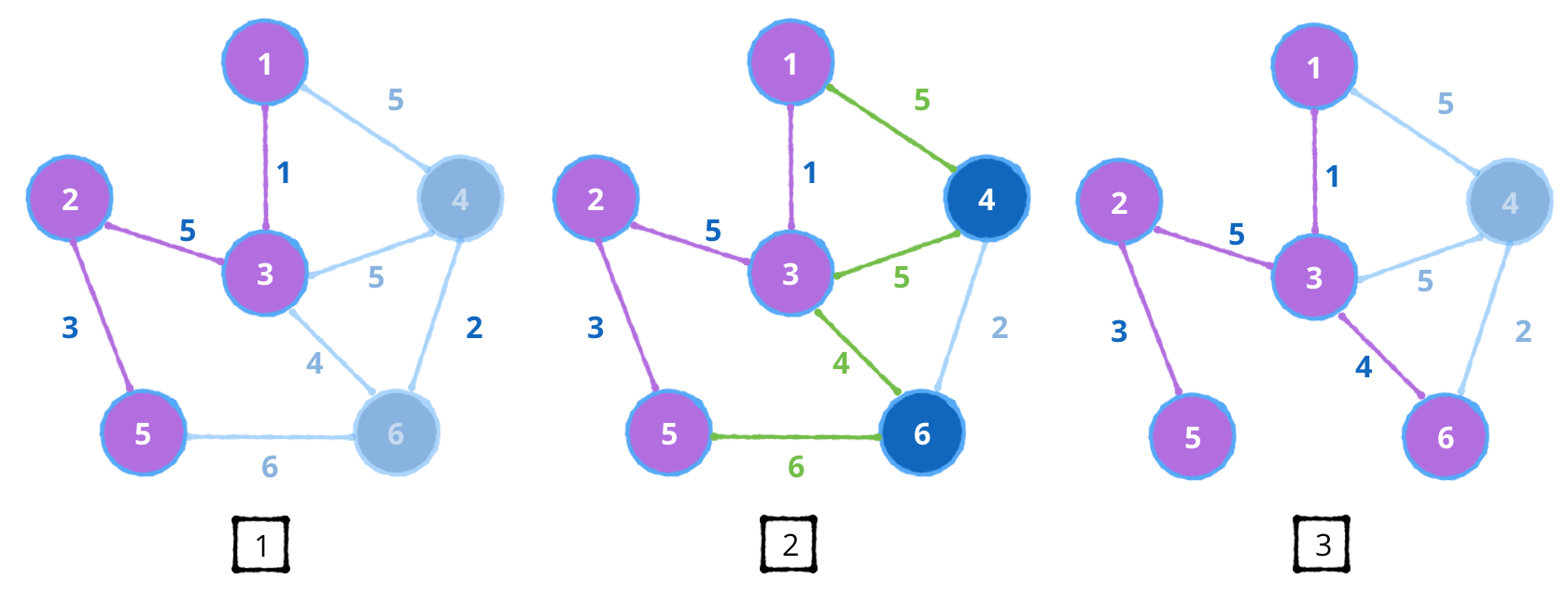1. You now have visited vertices { 2, 3, 5, 1 }.
2. Choose the shortest edge from these vertices. The next nearest edges are { 5, 5, 4, 6 } . You pick edge 4 since it’s the smallest, which routes to vertex 6.
3. Notice that edge 6 between vertex 5 and vertex 6 can be removed since these vertices have already been visited.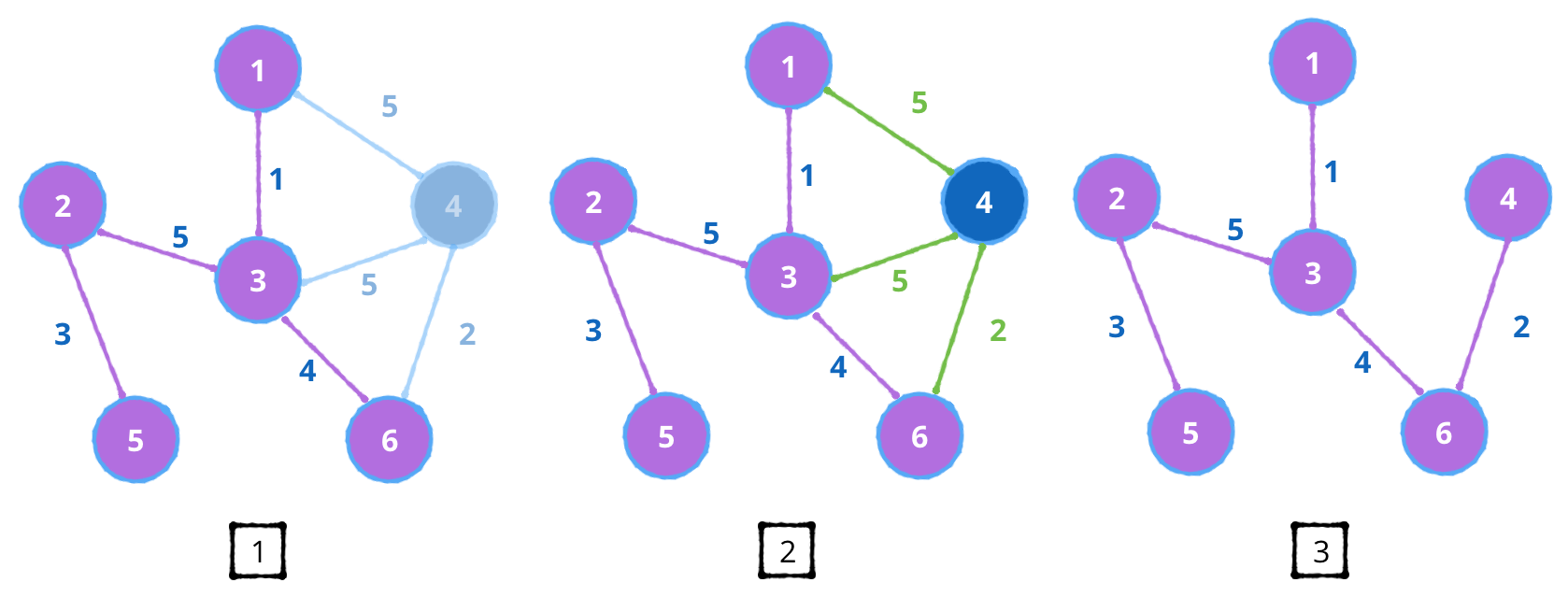1. Finally you now have visited vertices {2, 5, 3, 1, 6 }.
2. Choose the shortest edge from these vertices. The next nearest edges are { 5, 5, 2 } You pick edge 2 since it’s the smallest, which routes to vertex 4 .
3. Notice that the rest of the edges { 5, 5 } connected to vertex 4 from vertex 1 and vertex 3 can be removed since these vertices have been visited.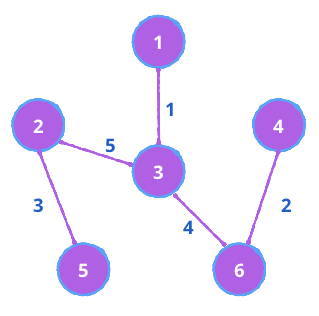RW Airways can now redirect their airplanes to fly these routes to cut cost! This means more profit! Are you starting to see the pattern here? Let’s move on to the implementation!

## ImplementationDownload the starter playground. Open the Project Navigator to see the list of files, and familiarize yourself with the Sources folder:

1. You will be using an adjacency list to create your graph network.
2. You will be using a Priority Queue (implemented using a Heap). This data structure will store edges adjacent to vertices you visit. The root of this heap will always have the smallest weighted edge.

Again, if you want more information on how these classes work, check out our Swift heap and priority queue tutorial.

## Setup

Navigate to the root Playground and add the following code:

```class Prim<T: Hashable> { // 1
typealias Graph = AdjacencyList<T> // 2
var priorityQueue = PriorityQueue<(vertex: Vertex<T>, weight: Double, parent: Vertex<T>?)>(
sort: { \$0.weight < \$1.weight }) // 3
}
```
1. You have declared a class named `Prim`, you want this algorithm to be generic, so it can find the minimum spanning tree of any type, given that it's `Hashable`.
2. This class will use the `AdjacencyList` to hold your graphs.
3. You define a minimum `priorityQueue`, where it holds the current vertex, the weight of the edge between the vertices, and the parent vertex.

Next add the following after `priorityQueue`:

```func produceMinimumSpanningTree(graph: Graph) -> (cost: Double, mst: Graph) { // 1
var cost = 0.0 // 2
let mst = Graph() // 3
var visited = Set<Vertex<T>>() // 4

return (cost: cost, mst: mst) // 5
}
```
1. Define a function `produceMinimumSpanningTree(_:)`, that takes a `Graph` network, and returns the minimum total cost of all edges, and the minimum spanning tree.
2. Define the cost variable, used to aggregate all weights.
3. Create a graph to construct your minimum spanning tree.
4. Create a `Set` to store all vertices visited.
5. Once prim's algorithm is complete, return the results.

## Initiate the algorithm

Add the following after the `visited` variable:

```guard let start = graph.getAllVertices().first else { // 1
return (cost: cost, mst: mst)
}
priorityQueue.enqueue((vertex: start, weight: 0.0, parent: nil)) //2
```
1. Start by picking any vertex. In this case, we grab all vertices from the adjacency list, and pick the first one.
2. Add the start vertex into the `priorityQueue`. In this case since you haven't visit any other vertex, the edge weight is zero, and the parent is `nil`.

## Step by Step and grab the smallest!

Next add the following code right after:

```while let head = priorityQueue.dequeue() { // 1
if visited.contains(vertex) { // 2
continue
}
visited.insert(vertex) // 3

if let prev = head.parent { // 5
}

if let neighbours = graph.edges(from: vertex) { // 6
for neighbour in neighbours { // 7
if !visited.contains(neighbour.destination) { // 8
priorityQueue.enqueue((vertex: neighbour.destination, weight: neighbour.weight ?? 0.0, parent: vertex))
}
}
}
}
```

This is the main part of prim's algorithm where you select the smallest edge every time you visit a vertex. Let's go over how the code works:

1. Check to see if there is a set of vertices and weight in the priority queue. If the queue is empty, prim's algorithm is complete.

Note: Every time a `(current vertex, weight, parent vertex)` set is dequeued from the priority queue, you are guaranteed that the weight between two vertices is always the minimum.

2. Check to see if the current vertex you are visiting has been visited before.
If it has been visited, restart the loop, and dequeue the next set of vertices and edge between them.
3. If it has been yet to visited, add it to the set of visited vertices
4. Add the weight between the set of vertices to the total cost.
5. Check to see if the current vertex you are visiting has a parent vertex. If there is, add it to the minimum spanning tree you are constructing.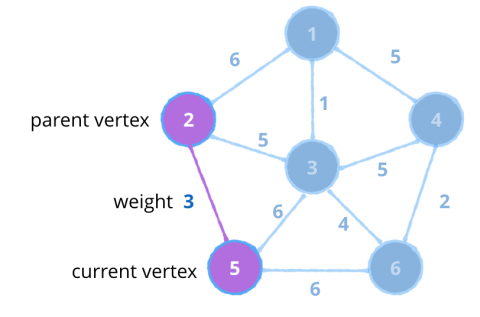6. Now go through all the neighbouring edges from the current vertex, and add it to the queue, as long as the destination vertex has yet to be visited.

That's all there is to it!

## Testing it out

Add the following code after `Prim` class declaration:

```var graph = AdjacencyList<Int>()
let one = graph.createVertex(data: 1)
let two = graph.createVertex(data: 2)
let three = graph.createVertex(data: 3)
let four = graph.createVertex(data: 4)
let five = graph.createVertex(data: 5)
let six = graph.createVertex(data: 6)

graph.add(.undirected, from: one, to: two, weight: 6)
graph.add(.undirected, from: one, to: three, weight: 1)
graph.add(.undirected, from: one, to: four, weight: 5)
graph.add(.undirected, from: two, to: three, weight: 5)
graph.add(.undirected, from: two, to: five, weight: 3)
graph.add(.undirected, from: three, to: four, weight: 5)
graph.add(.undirected, from: three, to: five, weight: 6)
graph.add(.undirected, from: three, to: six, weight: 4)
graph.add(.undirected, from: four, to: six, weight: 2)
graph.add(.undirected, from: five, to: six, weight: 6)

let prim = Prim<Int>()
let (cost, mst) = prim.produceMinimumSpanningTree(graph: graph)
print("cost: \(cost)")
print("mst:")
print(mst.description)
```

You should see a string representation of the example below: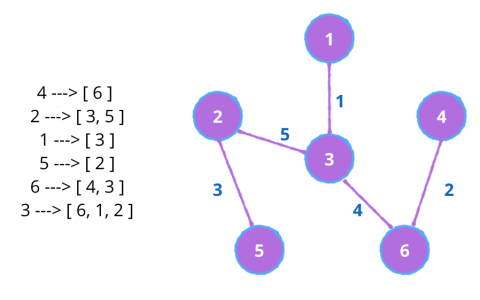## Where to go from here?

I hope you enjoyed this tutorial on prim's algorithm! Here is a playground with the above code. You can also find the original implementation and further discussion on the repo for prim's algorithm.

This was just one of the many algorithms in the Swift Algorithm Club repository. If you're interested in more, check out the repo. It's in your best interest to know about algorithms and data structures - they're solutions to many real-world problems, and are frequently asked as interview questions. Plus it's fun!

Stay tuned for many more tutorials from the Swift Algorithm club in the future. In the meantime, if you have any questions on implementing trees in Swift, please join the forum discussion below.

Note: The Swift Algorithm Club is always looking for more contributors. If you've got an interesting data structure, algorithm, or even an interview question to share, don't hesitate to contribute! To learn more about the contribution process, check out our Join the Swift Algorithm Club article.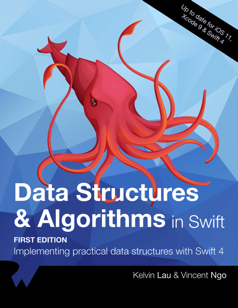If you enjoyed what you learned in this tutorial, why not check out our Data Structures and Algorithms in Swift book, available on our store?

In Data Structures and Algorithms in Swift, you’ll learn how to implement the most popular and useful data structures and when and why you should use one particular datastructure or algorithm over another. This set of basic data structures and algorithms will serve as an excellent foundation for building more complex and special-purpose constructs.

As well, the high-level expressiveness of Swift makes it an ideal choice for learning these core concepts without sacrificing performance.

• You’ll start with the fundamental structures of linked lists, queues and stacks, and see how to implement them in a highly Swift-like way.
• Move on to working with various types of trees, including general purpose trees, binary trees, AVL trees, binary search trees and tries.
• Go beyond bubble and insertion sort with better-performing algorithms, including mergesort, radix sort, heap sort and quicksort.
• Learn how to construct directed, non-directed and weighted graphs to represent many real-world models, and traverse graphs and trees efficiently with breadth-first, depth-first, Dijkstra’s and Prim’s algorithms to solve problems such as finding the shortest path or lowest cost in a network.
• And much, much more!

By the end of this book, you’ll have hands-on experience solving common issues with data structures and algorithms — and you’ll be well on your way to developing your own efficient and useful implementations.